Check Full Chapter Explained - Continuity and Differentiability - Application of Derivatives (AOD) Class 12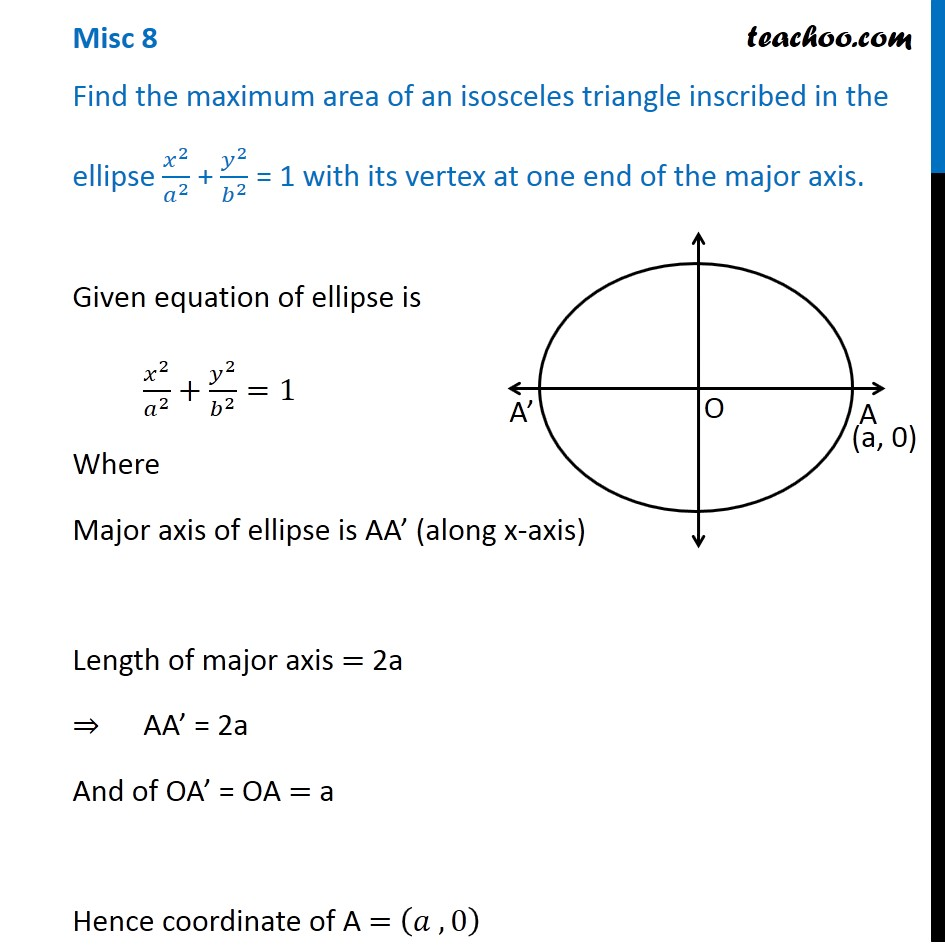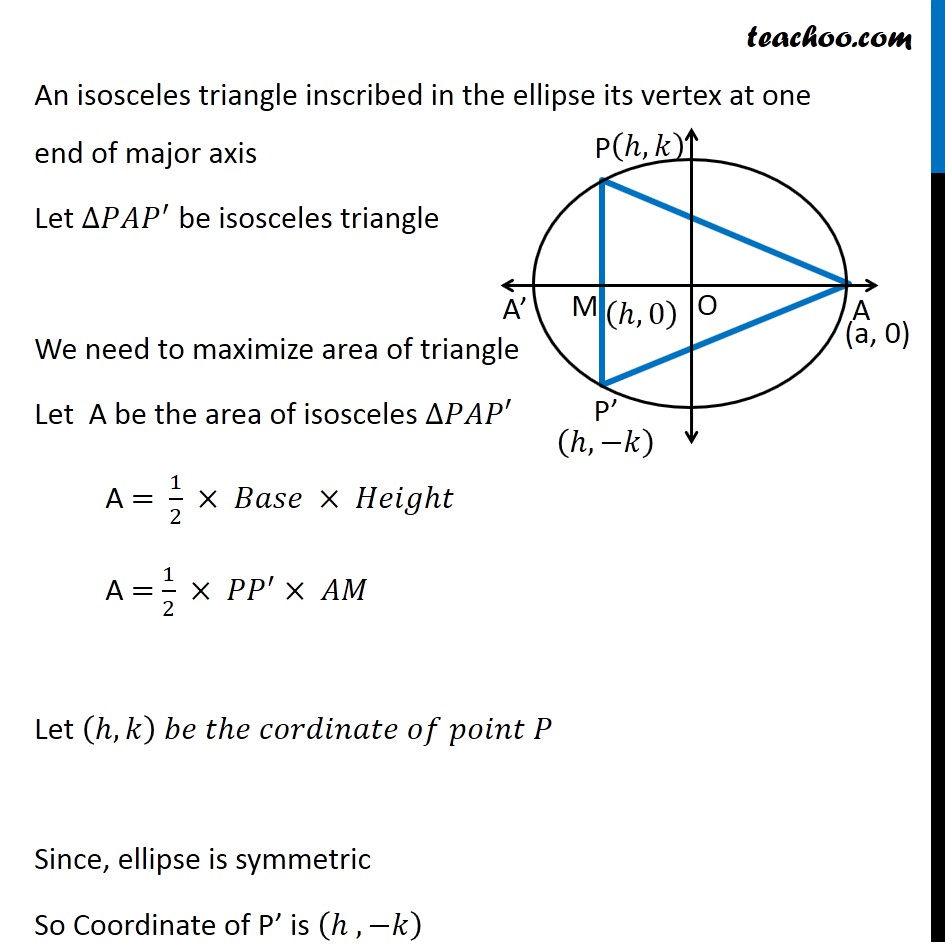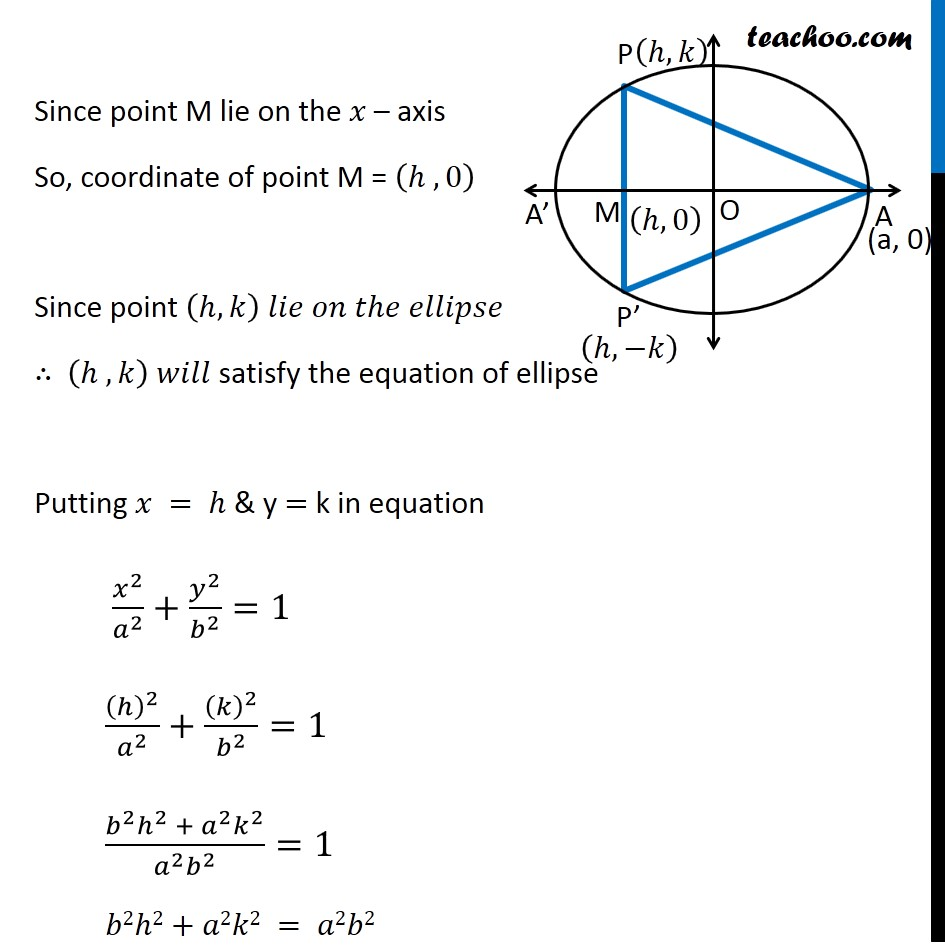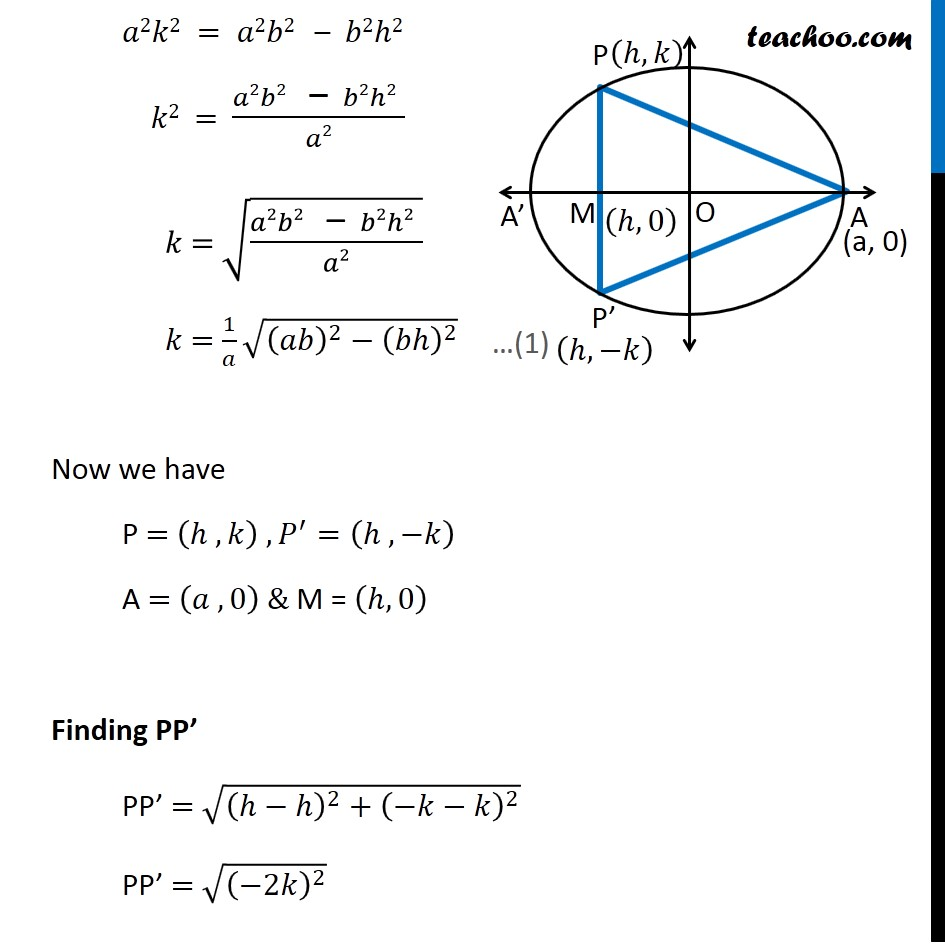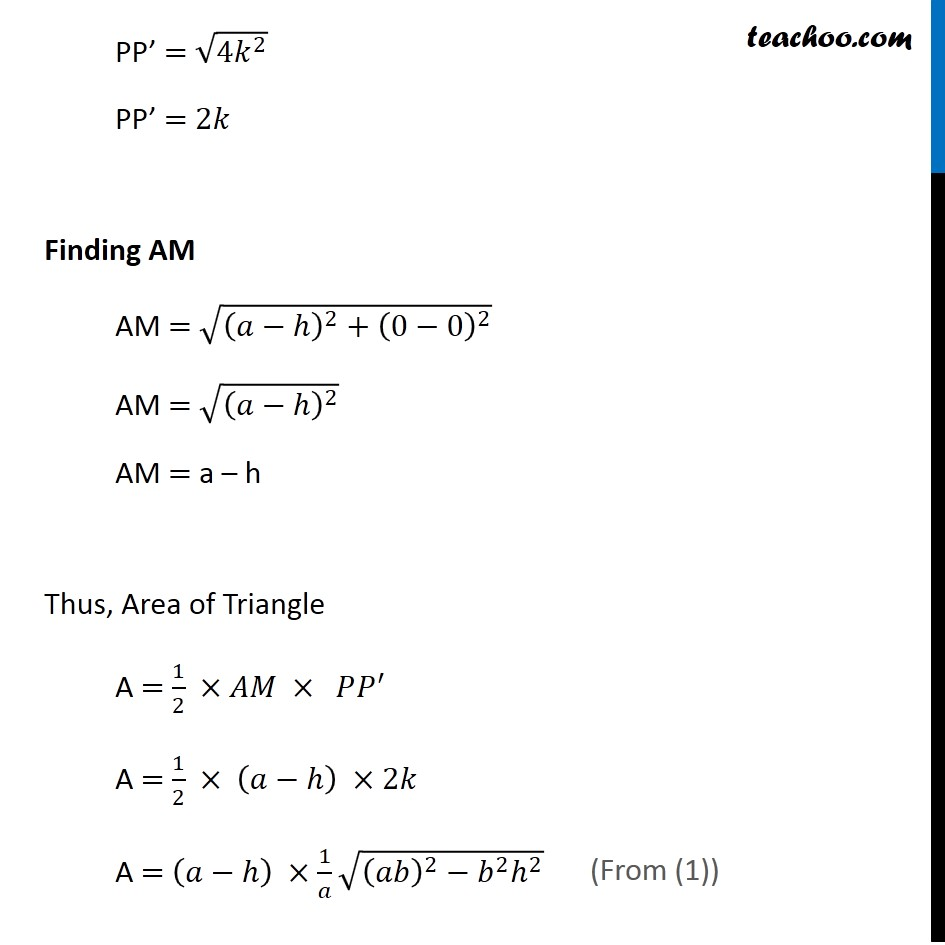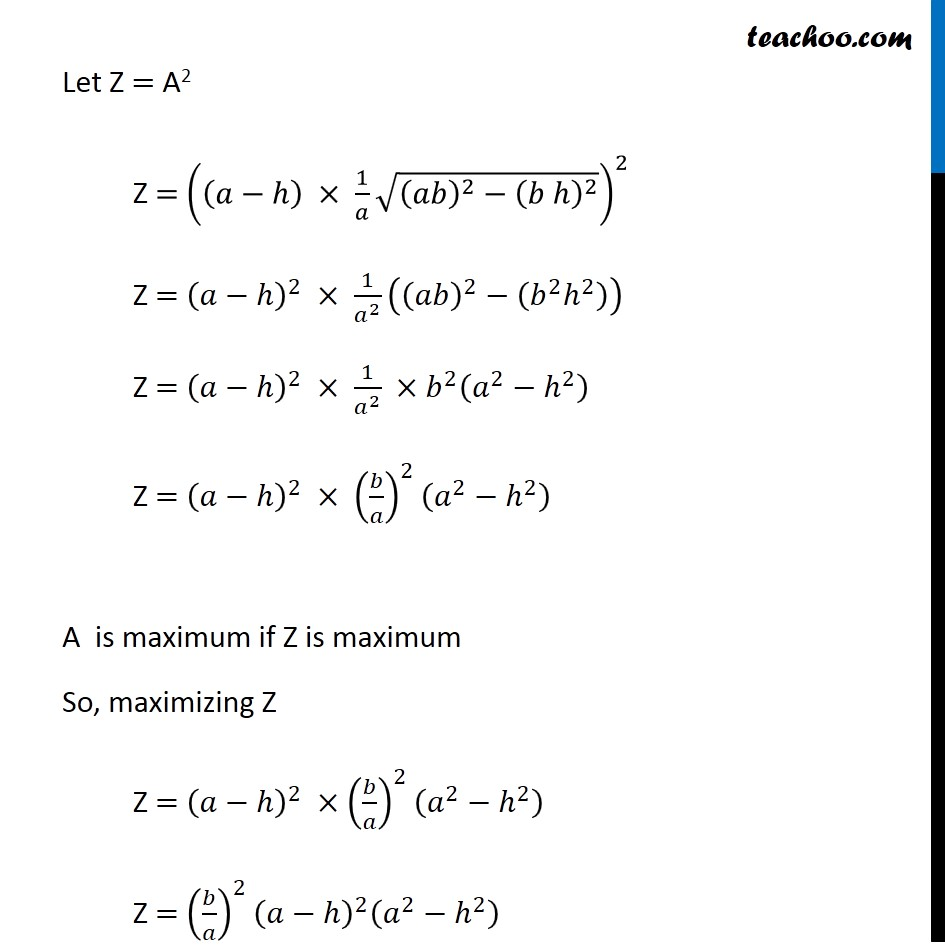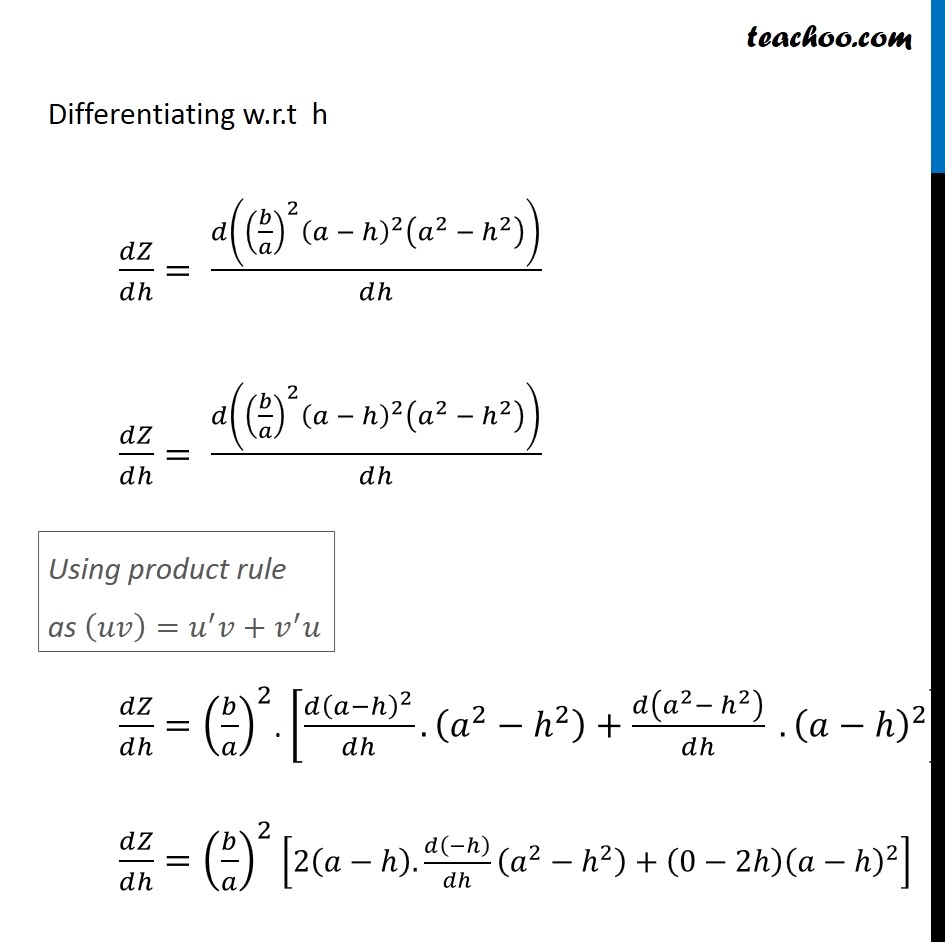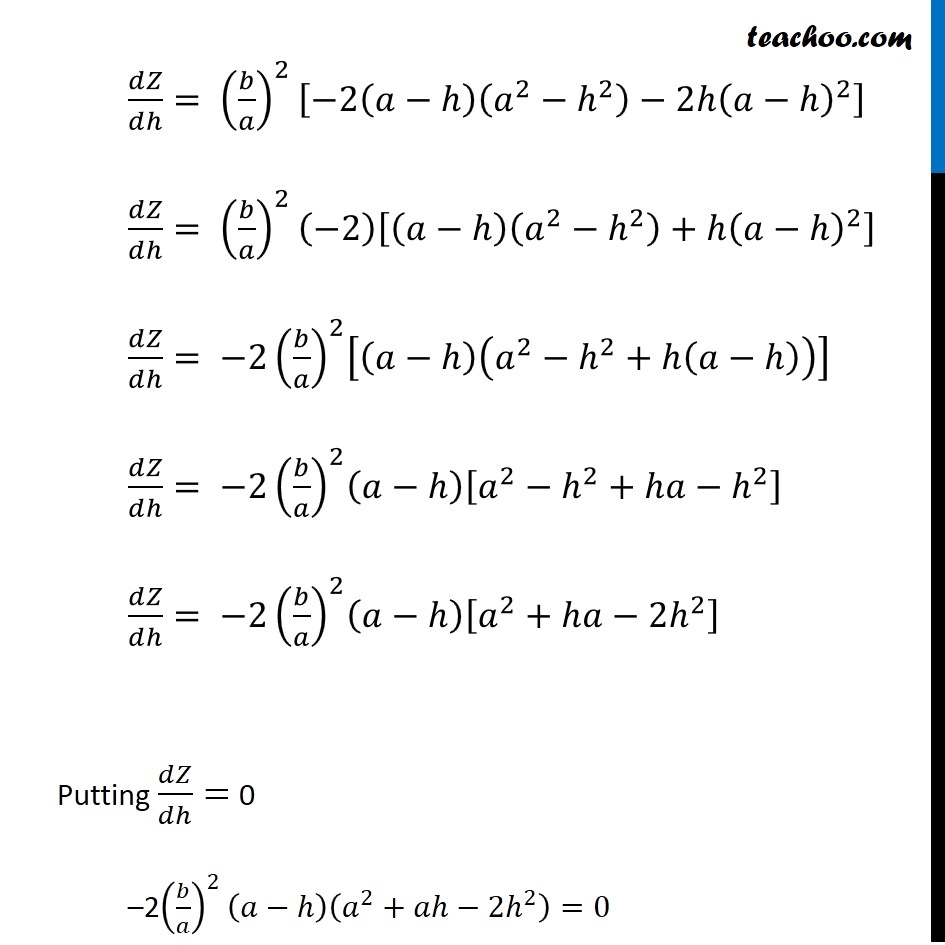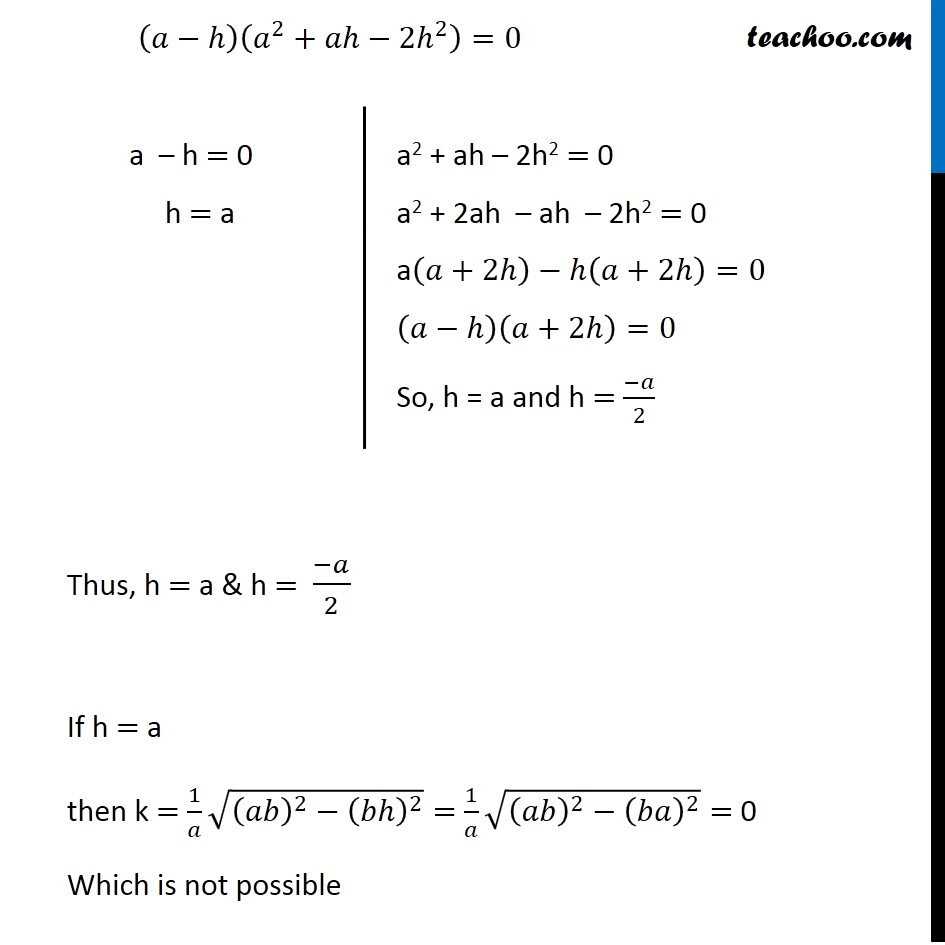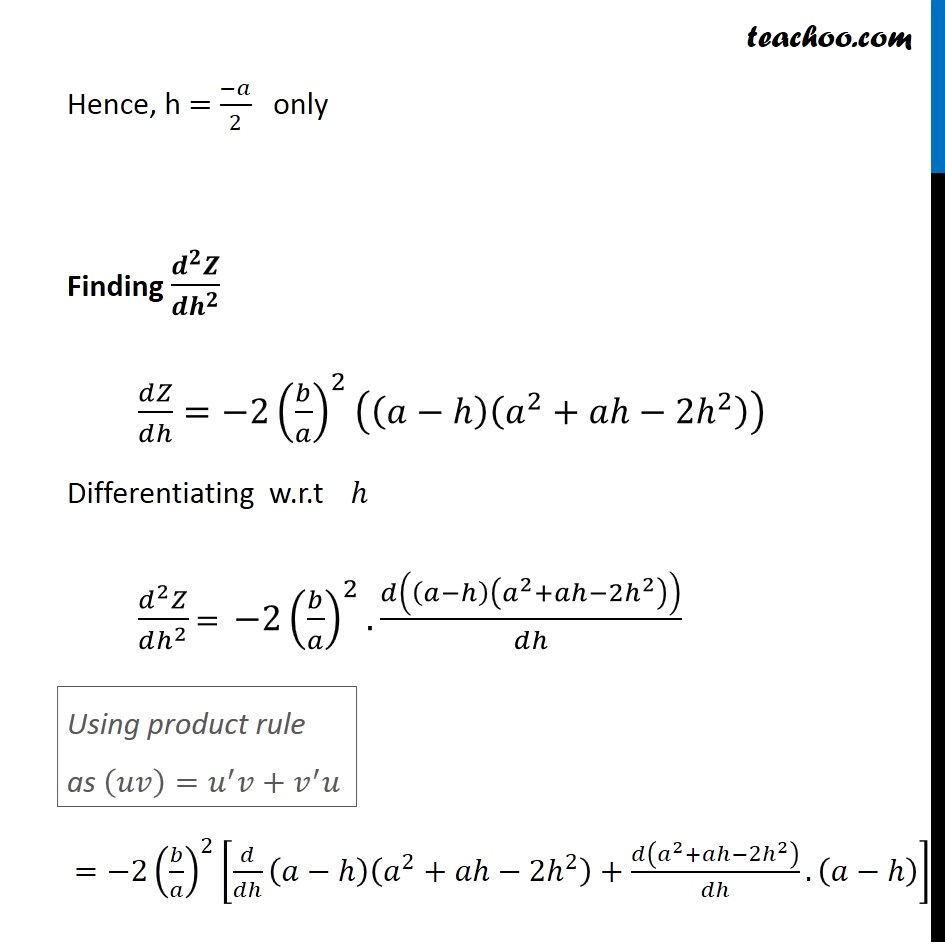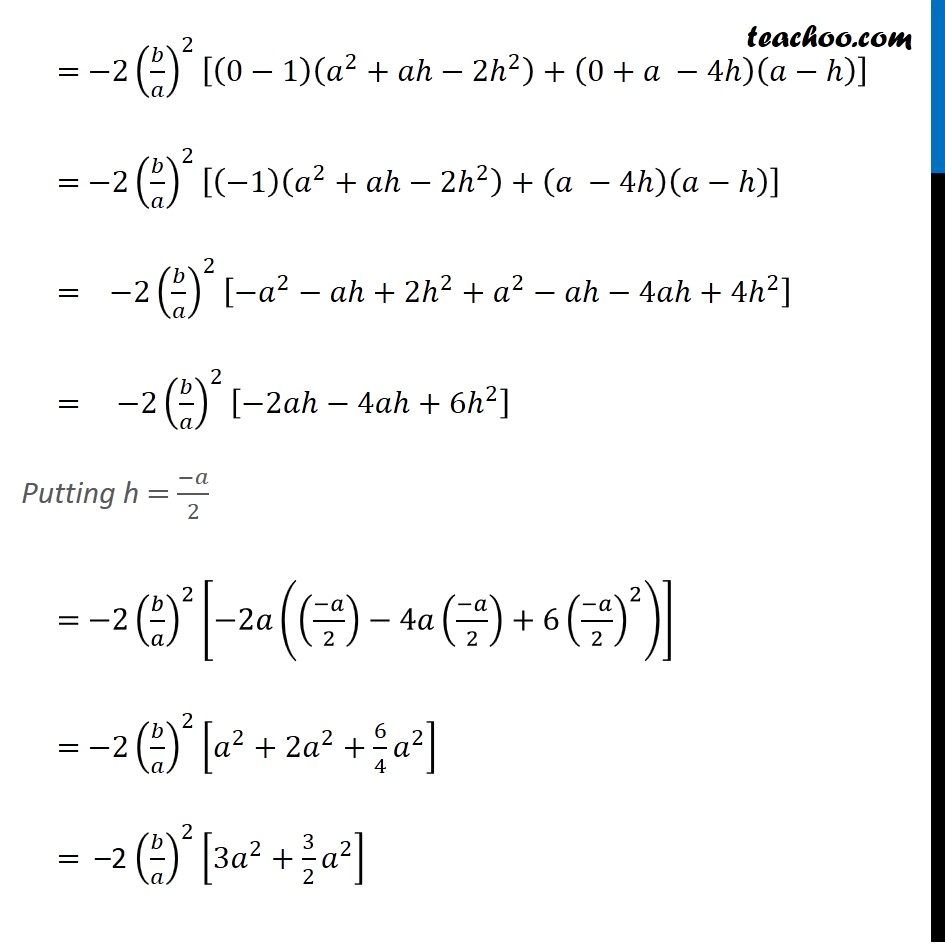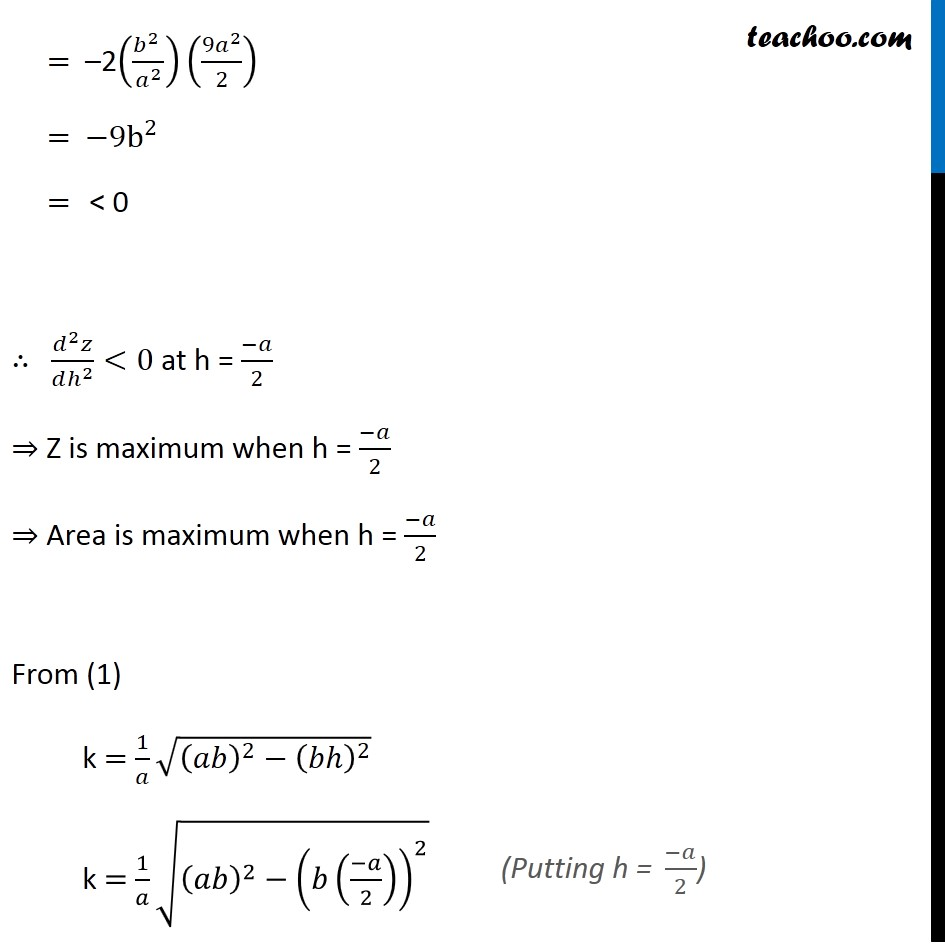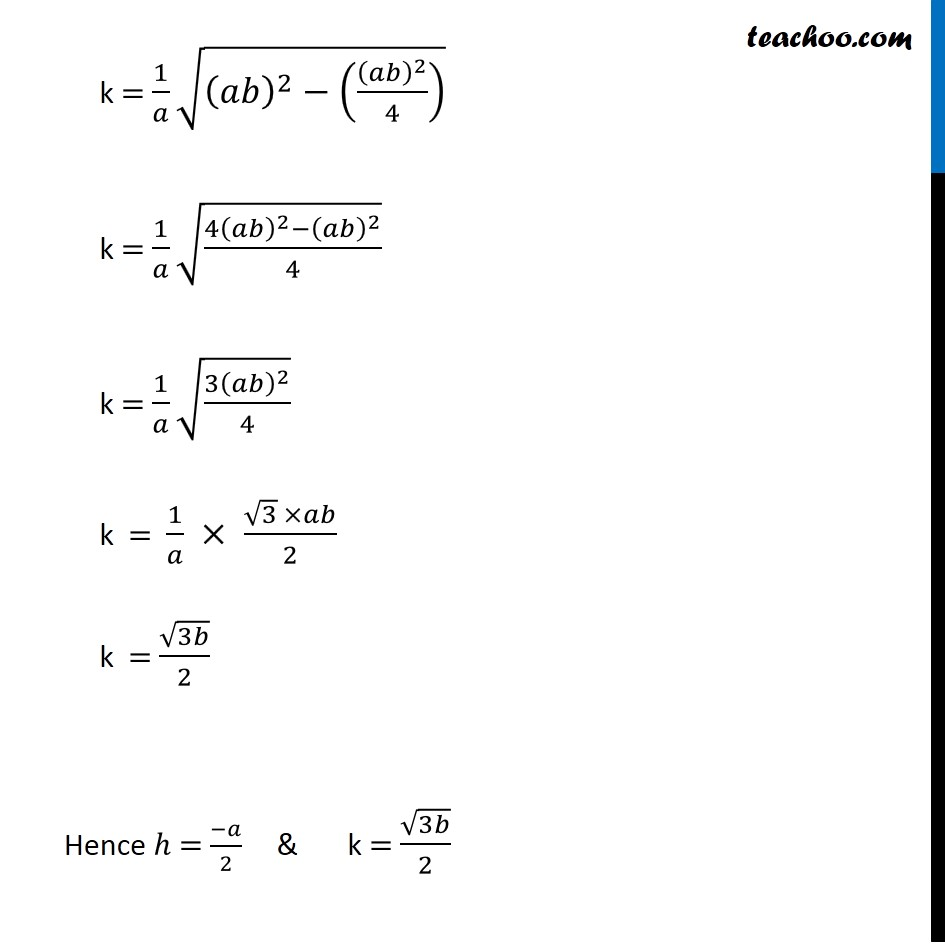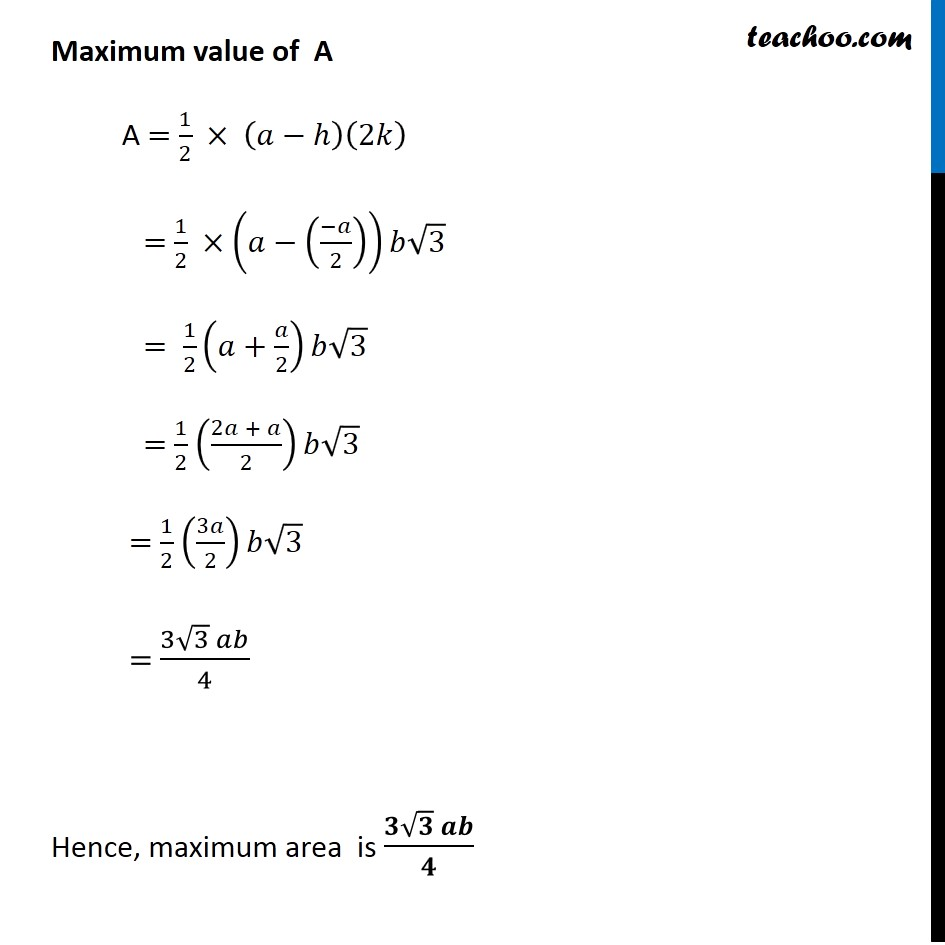1. Chapter 6 Class 12 Application of Derivatives
2. Serial order wise
3. Miscellaneous

Transcript

Misc 8 Find the maximum area of an isosceles triangle inscribed in the ellipse 𝑥^2/𝑎^2 + 𝑦^2/𝑏^2 = 1 with its vertex at one end of the major axis. Given equation of ellipse is 𝑥^2/𝑎^2 +𝑦^2/𝑏^2 =1 Where Major axis of ellipse is AA’ (along x-axis) Length of major axis = 2a ⇒ AA’ = 2a And of OA’ = OA = a Hence coordinate of A = (𝑎 , 0) An isosceles triangle inscribed in the ellipse its vertex at one end of major axis Let ∆𝑃𝐴𝑃^′ be isosceles triangle We need to maximize area of triangle Let A be the area of isosceles ∆𝑃𝐴𝑃′ A = 1/2 × 𝐵𝑎𝑠𝑒 × 𝐻𝑒𝑖𝑔ℎ𝑡 A = 1/2 × 〖𝑃𝑃〗^′× 𝐴𝑀 Let (ℎ, 𝑘) 𝑏𝑒 𝑡ℎ𝑒 𝑐𝑜𝑟𝑑𝑖𝑛𝑎𝑡𝑒 𝑜𝑓 𝑝𝑜𝑖𝑛𝑡 𝑃 Since, ellipse is symmetric So Coordinate of P’ is (ℎ , −𝑘) Since point M lie on the 𝑥 – axis So, coordinate of point M = (ℎ , 0) Since point (ℎ, 𝑘) 𝑙𝑖𝑒 𝑜𝑛 𝑡ℎ𝑒 𝑒𝑙𝑙𝑖𝑝𝑠𝑒 ∴ (ℎ , 𝑘) 𝑤𝑖𝑙𝑙 satisfy the equation of ellipse Putting 𝑥 = ℎ & y = k in equation 𝑥^2/𝑎^2 +𝑦^2/𝑏^2 =1 (ℎ)^2/𝑎^2 +(𝑘)^2/𝑏^2 =1 (𝑏^2 ℎ^2 + 𝑎^2 𝑘^2)/(𝑎^2 𝑏^2 )=1 𝑏2ℎ2+𝑎2𝑘2 = 𝑎2𝑏2 𝑎2𝑘2 = 𝑎2𝑏2 – 𝑏2ℎ2 𝑘2 = (𝑎2𝑏2" – " 𝑏2ℎ2" " )/𝑎2 𝑘 = √((𝑎2𝑏2" – " 𝑏2ℎ2" " )/𝑎2) 𝑘 = 1/𝑎 √((𝑎𝑏)^2−(𝑏ℎ)^2 ) Now we have P = (ℎ , 𝑘) , 𝑃^′=(ℎ , −𝑘) A = (𝑎 , 0) & M = (ℎ,0) Finding PP’ PP’ = √((ℎ−ℎ)^2+(−𝑘−𝑘)^2 ) PP’ = √((−2𝑘)^2 ) PP’ = √(〖4𝑘〗^2 ) PP’ = 2𝑘 Finding AM AM = √((𝑎−ℎ)^2+(0−0)^2 ) AM = √((𝑎−ℎ)^2 ) AM = a – h Thus, Area of Triangle A = 1/2 ×𝐴𝑀 × 𝑃𝑃^′ A = 1/2 × (𝑎−ℎ) ×2𝑘 A = (𝑎−ℎ) ×1/𝑎 √((𝑎𝑏)^2−𝑏^2 ℎ^2 ) Let Z = A2 Z = ((𝑎−ℎ) × 1/𝑎 √((𝑎𝑏)^2−(𝑏 ℎ)^2 ))^2 Z = (𝑎−ℎ)^2 × 1/𝑎^2 ((𝑎𝑏)^2−(𝑏^2 ℎ^2 )) Z = (𝑎−ℎ)^2 × 1/𝑎^2 ×𝑏^2 (𝑎^2−ℎ^2 ) Z = (𝑎−ℎ)^2 × (𝑏/𝑎)^2 (𝑎^2−ℎ^2 ) A is maximum if Z is maximum So, maximizing Z Z = (𝑎−ℎ)^2 ×(𝑏/𝑎)^2 (𝑎^2−ℎ^2 ) Z = (𝑏/𝑎)^2 (𝑎−ℎ)^2 (𝑎^2−ℎ^2 ) Differentiating w.r.t h 𝑑𝑍/𝑑ℎ= 𝑑((𝑏/𝑎)^2 (𝑎 − ℎ)^2 (𝑎^2 − ℎ^2 ))/𝑑ℎ 𝑑𝑍/𝑑ℎ= 𝑑((𝑏/𝑎)^2 (𝑎 − ℎ)^2 (𝑎^2 − ℎ^2 ))/𝑑ℎ 𝑑𝑍/𝑑ℎ=(𝑏/𝑎)^2. [(𝑑(𝑎−ℎ)^2)/𝑑ℎ.(𝑎^2−ℎ^2 )+𝑑(𝑎^2− ℎ^2 )/𝑑ℎ . (𝑎−ℎ)^2 ] 𝑑𝑍/𝑑ℎ=(𝑏/𝑎)^2 [2(𝑎−ℎ). 𝑑(−ℎ)/𝑑ℎ (𝑎^2−ℎ^2 )+(0−2ℎ) (𝑎−ℎ)^2 ] 𝑑𝑍/𝑑ℎ= (𝑏/𝑎)^2 [−2(𝑎−ℎ)(𝑎^2−ℎ^2 )−2ℎ(𝑎−ℎ)^2 ] 𝑑𝑍/𝑑ℎ= (𝑏/𝑎)^2 (−2)[(𝑎−ℎ)(𝑎^2−ℎ^2 )+ℎ(𝑎−ℎ)^2 ] 𝑑𝑍/𝑑ℎ= 〖−2(𝑏/𝑎)〗^2 [(𝑎−ℎ)(𝑎^2−ℎ^2+ℎ(𝑎−ℎ))] 𝑑𝑍/𝑑ℎ= 〖−2(𝑏/𝑎)〗^2 (𝑎−ℎ)[𝑎^2−ℎ^2+ℎ𝑎−ℎ^2 ] 𝑑𝑍/𝑑ℎ= 〖−2(𝑏/𝑎)〗^2 (𝑎−ℎ)[𝑎^2+ℎ𝑎−2ℎ^2 ] Putting 𝑑𝑍/𝑑ℎ= 0 –2(𝑏/𝑎)^2 (𝑎−ℎ)(𝑎^2+𝑎ℎ−2ℎ^2 )=0 (𝑎−ℎ)(𝑎^2+𝑎ℎ−2ℎ^2 )=0 Thus, h = a & h = (−𝑎)/2 If h = a then k = 1/𝑎 √((𝑎𝑏)^2−(𝑏ℎ)^2 )= 1/𝑎 √((𝑎𝑏)^2−(𝑏𝑎)^2 )= 0 Which is not possible a – h = 0 h = a a2 + ah – 2h2 = 0 a2 + 2ah – ah – 2h2 = 0 a(𝑎+2ℎ)−ℎ(𝑎+2ℎ)=0 (𝑎−ℎ)(𝑎+2ℎ)=0 So, h = a and h = (−𝑎)/2 Hence, h = (−𝑎)/2 only Finding (𝒅^𝟐 𝒁)/(𝒅𝒉^𝟐 ) 𝑑𝑍/𝑑ℎ=−2(𝑏/𝑎)^2 ((𝑎−ℎ)(𝑎^2+𝑎ℎ−2ℎ^2 )) Differentiating w.r.t ℎ (𝑑^2 𝑍)/(𝑑ℎ^2 ) = −2(𝑏/𝑎)^2. 𝑑((𝑎−ℎ)(𝑎^2+𝑎ℎ−2ℎ^2 ))/𝑑ℎ =−2(𝑏/𝑎)^2 [𝑑/𝑑ℎ (𝑎−ℎ)(𝑎^2+𝑎ℎ−2ℎ^2 )+𝑑(𝑎^2+𝑎ℎ−2ℎ^2 )/𝑑ℎ.(𝑎−ℎ)] Using product rule as (𝑢𝑣)=𝑢^′ 𝑣+𝑣^′ 𝑢 = −2(𝑏/𝑎)^2 [(0−1)(𝑎^2+𝑎ℎ−2ℎ^2 )+(0+𝑎 −4ℎ)(𝑎−ℎ)] = −2(𝑏/𝑎)^2 [(−1)(𝑎^2+𝑎ℎ−2ℎ^2 )+(𝑎 −4ℎ)(𝑎−ℎ)] = −2(𝑏/𝑎)^2 [−𝑎^2−𝑎ℎ+2ℎ^2+𝑎^2−𝑎ℎ−4𝑎ℎ+4ℎ^2 ] = −2(𝑏/𝑎)^2 [−2𝑎ℎ−4𝑎ℎ+6ℎ^2 ] Putting h = (−𝑎)/2 = −2(𝑏/𝑎)^2 [−2𝑎(((−𝑎)/2)−4𝑎((−𝑎)/2)+6((−𝑎)/2)^2 )] = −2(𝑏/𝑎)^2 [𝑎^2+2𝑎^2+6/4 𝑎^2 ] = –2 (𝑏/𝑎)^2 [3𝑎^2+3/2 𝑎^2 ] = –2((𝑏^2 )/𝑎^2 )((9𝑎^2)/2) = −9b^2 = < 0 ∴ (𝑑^2 𝑧)/(𝑑ℎ^2 )<0 at h = (−𝑎)/2 ⇒ Z is maximum when h = (−𝑎)/2 ⇒ Area is maximum when h = (−𝑎)/2 From (1) k = 1/𝑎 √((𝑎𝑏)^2−(𝑏ℎ)^2 ) k = 1/𝑎 √((𝑎𝑏)^2−(𝑏((−𝑎)/2))^2 ) ("Putting h = " (−𝑎)/2) k = 1/𝑎 √((𝑎𝑏)^2−((𝑎𝑏)^2/4) ) k = 1/𝑎 √((4(𝑎𝑏)^2−(𝑎𝑏)^2)/4) k = 1/𝑎 √((3(𝑎𝑏)^2)/4) k = 1/𝑎 × (√3 ×𝑎𝑏)/2 k = √3𝑏/2 Hence ℎ = (−𝑎)/2 & k = √3𝑏/2 Maximum value of A A = 1/2 × (𝑎−ℎ)(2𝑘) = 1/2 ×(𝑎−((−𝑎)/2))𝑏√3 = 1/2 (𝑎+𝑎/2)𝑏√3 = 1/2 ((2𝑎 + 𝑎)/2)𝑏√3 = 1/2 (3𝑎/2)𝑏√3 = (3√3 𝑎𝑏)/4 Hence, maximum area is (𝟑√𝟑 𝒂𝒃)/𝟒

Miscellaneous